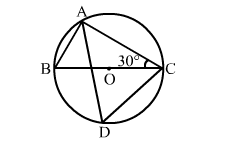# In the given figure, BOC is a diameter of a circle with centre O

Question:

In the given figure, BOC is a diameter of a circle with centre O. If BCA = 30°, then ∠CDA = ?
(a) 30°
(b) 45°
(c) 60°
(d) 50°Solution:

(c) 60°
Angles in a semi circle measure 90°.
∴ ∠BAC = 90°
In
Δ ABC, we have:
∠BAC + ∠ABC + ∠BCA = 180° (Angle sum property of a triangle)
∴ 90° + ∠ABC + 30° = 180°
⇒ ∠ABC = (180° - 120°) = 60°
∴ ∠CDA = ∠ABC = 60° (Angles in the same segment of a circle)